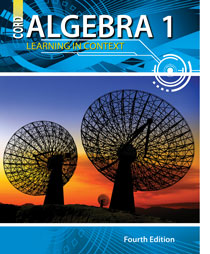# Algebra 1 - 4th Edition

## Chapter 10: Rational Expressions and Equations

Some links are repeated for use with more than one lesson.

### 10.1 Graphing Rational Expressions

http://www.sparknotes.com/math/algebra2/rationalexpressions/
This site contains links to pages about rational expression terms and problems, as well as adding, subtracting, multiplying, and dividing rational expressions.

http://www.analyzemath.com/Rational_expressions/Rational_express.html

http://www.mathsisfun.com/algebra/rational-expression.html
This site presents and provides practice for graphing and analyzing rational expressions.

http://www.algebralab.org/lessons/lesson.aspx?file=algebra_rational_graphing.xml
This site presents guidelines and practice problems for graphing rational expressions.

### 10.2 Simplifying Rational Expressions

http://www.purplemath.com/modules/rtnldefs2.htm
This series of pages explains how to simplify rational expressions.

http://www.algebralab.org/lessons/lesson.aspx?file=Algebra_rational_simplifying.xml
This site has notes and examples on how to simplify rational expressions.

### 10.3 Multiplying and Dividing Rational Expressions

http://www.purplemath.com/modules/rtnlmult.htm

http://www.purplemath.com/modules/rtnlmult2.htm

### 10.4 Adding and Subtracting Rational Expressions

This site demonstrates how to add and subtract rational expressions through examples.

This page has notes on how to add and subtract rational expressions and has step-by-step solved examples.

### 10.5 Inverse Variation

http://www.explorelearning.com/index.cfm?method=cResource.dspDetail&ResourceID=129
This page contains a gizmo that has a graph displaying direct and inverse variation.

http://www.sparknotes.com/math/algebra1/variation/section2.rhtml